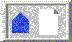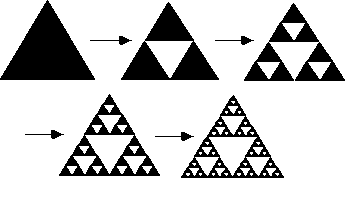Why does the Sierpinski triangle (Next Section)Chaos in the Classroom (Cover Page)The Chaos Game (Previous Section)BU Math Home Page

# The Sierpinski triangle.

The Sierpinski triangle S may also be constructed using a deterministic rather than a random algorithm. To see this, we begin with any triangle. Then we use the midpoints of each side as the vertices of a new triangle, which we then remove from the original. This leaves us with three triangles, each of which has dimensions exactly one-half the dimensions of the original triangle, and area exactly one-fourth of the original area. Also, each remaining triangle is similar to the original.

Now we continue (or iterate) this process. From each remaining triangle we remove the "middle" leaving behind three smaller triangles each of which has dimensions one-half of those of the parent triangle (and one-fourth of the original triangle). Clearly, 9 triangles remain at this stage. At the next iteration, 27 small triangles, then 81, and, at the Nth stage, 3^N small triangles remain. It is easy to check that the dimensions of the triangles that remain after the Nth iteration are exactly 1/2^N of the original dimensions. See Figure 3.Figure: Deterministic construction of SWhy does the Sierpinski triangle (Next Section)Chaos in the Classroom (Cover Page)The Chaos Game (Previous Section)BU Math Home Page

Robert L. Devaney
Sun Apr 2 14:31:18 EDT 1995